Next: MISSING DATA AND UNKNOWN Up: MISSING DATA IN ONE Previous: MISSING DATA IN ONE

## Missing-data program

There are two ways to code the missing-data estimation, one conceptually simple and the other leading to a concise program. Begin with a given filterand create a shifted-column matrix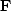,as in equation. The problem is that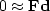whereis the data. The columns ofare of two types, those that multiply missing data values and those that multiply known data values. Suppose we reorganizeinto two collections of columns: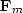for the missing data values, and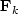for the known data values. Now, instead of, we have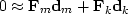or.Taking,we have simply an overdetermined set of simultaneous equations like,which we solved with cgmeth().

The trouble with this approach is that it is awkward to program the partitioning of the operator into the known and missing parts, particularly if the application of the operator uses arcane techniques, such as those used by the fast Fourier transform operator or various numerical approximations to differential or partial differential operators that depend on regular data sampling. Even for the modest convolution operator, we already have a library of convolution programs that handle a variety of end effects, and it would be much nicer to use the library as it is rather than recode it for all possible geometrical arrangements of missing data values. Here I take the main goal to be the clarity of the code, not the efficiency or accuracy of the solution. (So, if your problem consumes too many resources, and if you have many more known points than missing ones, maybe you should solve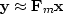and ignore what I suggest below.)

How then can we mimic the erratically structuredoperator using theoperator? When we multiply any vector into, we must be sure that the vector has zero-valued components to hit the columns ofthat correspond to missing data. When we look at the result of multiplying the adjointinto any vector, we must be sure to ignore the output at the rows corresponding to the missing data. As we will see, both of these criteria can be met using a single loop.

The missing-data program begins by loading the negative-filtered known data into a residual. Missing data should try to reduce this residual. The iterations proceed as in cgmeth(), invstack(), deghost(), shaper(), and iner(). The new ingredient in the missing-data subroutine miss1()is the simple constraint that the known data cannot be changed. Thus, after the gradient is computed, the components that correspond to known data values are set to zero.

```# fill in missing data on 1-axis by minimizing power out of a given filter.
#
subroutine miss1(     na, a, np, p, copy, niter)
integer iter, ip, nr, na,    np,          niter
real    p(np)           # in: known data with zeros for missing values.
# out: known plus missing data.
real    copy(np)        # in: copy(ip) vanishes where p(ip) is a missing value.
real    a(na)           # in: roughening filter
temporary real  dp(np),sp(np),  r(np+na-1),dr(np+na-1),sr(np+na-1)
nr = np+na-1
call contran( 0, 0, na,a, np, p, r)     # r = a*p   convolution
call scaleit  (        -1., nr,    r)     # r = -r
do iter= 0, niter {                             # niter= number missing or less
call contran( 1, 0, na,a, np,dp, r)     # dp(a,r)   correlation
do ip= 1, np
if( copy(ip) != 0.)             # missing data where copy(ip)==0
dp(ip) = 0.             # can't change known data
call contran( 0, 0, na,a, np,dp, dr)    # dr=a*dp   convolution
call cgstep( iter, np,p,dp,sp, nr,r,dr,sr)      # p=p+dp; r=r-dr
}
return; end
```

That prevents changes to the known data by motion any distance along the gradient. Likewise, motion along previous steps cannot perturb the known data values. Hence, the CG method (finding the minimum power in the plane spanned by the gradient and the previous step) leads to minimum power while respecting the constraints.

## EXERCISES:

1. Figure 2-6 seem to extrapolate to vanishing signals at the side boundaries. Why is that so, and what could be done to leave the sides unconstrained in that way?
2. Compare Figure 7 to the interpolation values you expect for the filter (1,0,-.5).
3. Indicate changes to miss1()for missing data in two dimensions.
4. Suppose the call in miss1()was changed from contran()to convin(). Predict the changed appearance of Figure 2.
5. Suppose the call in miss1() was changed from contran()to convin(). What other changes need to be made?
6. Show that the interpolation curve in Figure 3 is not parabolic as it appears, but cubic. (HINT: Show that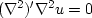.)
7. Verify by a program example that the number of iterations required with simple constraints is the number of free parameters. 1Next: MISSING DATA AND UNKNOWN Up: MISSING DATA IN ONE Previous: MISSING DATA IN ONE
Stanford Exploration Project
10/21/1998